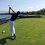Recently, on one of my math competitions, there was a problem that no one got, in clouding the teacher. So I was wondering if it's even answerable.

#### A number n has 4 distinct prime factors. Find the number of positive integers that divide n with remainder.

N-16.

194Note by Trevor Arashiro
6 years, 7 months ago

This discussion board is a place to discuss our Daily Challenges and the math and science related to those challenges. Explanations are more than just a solution — they should explain the steps and thinking strategies that you used to obtain the solution. Comments should further the discussion of math and science.

When posting on Brilliant:

• Use the emojis to react to an explanation, whether you're congratulating a job well done , or just really confused .
• Ask specific questions about the challenge or the steps in somebody's explanation. Well-posed questions can add a lot to the discussion, but posting "I don't understand!" doesn't help anyone.
• Try to contribute something new to the discussion, whether it is an extension, generalization or other idea related to the challenge.

MarkdownAppears as
*italics* or _italics_ italics
**bold** or __bold__ bold
- bulleted- list
• bulleted
• list
1. numbered2. list
1. numbered
2. list
Note: you must add a full line of space before and after lists for them to show up correctly
paragraph 1paragraph 2

paragraph 1

paragraph 2

[example link](https://brilliant.org)example link
> This is a quote
This is a quote
    # I indented these lines
# 4 spaces, and now they show
# up as a code block.

print "hello world"
# I indented these lines
# 4 spaces, and now they show
# up as a code block.

print "hello world"
MathAppears as
Remember to wrap math in $$ ... $$ or $ ... $ to ensure proper formatting.
2 \times 3 $2 \times 3$
2^{34} $2^{34}$
a_{i-1} $a_{i-1}$
\frac{2}{3} $\frac{2}{3}$
\sqrt{2} $\sqrt{2}$
\sum_{i=1}^3 $\sum_{i=1}^3$
\sin \theta $\sin \theta$
\boxed{123} $\boxed{123}$

Sort by:

Doesn't this depend on the multiplicity of the distinct prime factors?

Are we looking at $n = p_{1}^{a_{1}} * p_{2}^{a_{2}} * p_{3}^{a_{3}} * p_{4}^{a_{4}}$ for distinct primes $p_{k}$ with exponents $a_{k} \ge 1$?

If so, then the I think the desired number is $\prod_{k=1}^4 (a_{k} + 1)$.

- 6 years, 7 months ago

Sorry, but I just realized I worded the question slightly wrong. Its supposed to be "with remainder". So for this case, its just $n-\prod_{k=1}^4(a_k+1)$

But that's what I was thinking as well. Unfortunately, it's supposedly not the answer

- 6 years, 7 months ago

Weird. Is there any chance that the source of the supposed answer is incorrect? I see now how the "n - 16" and "194" answers arose; you are looking at $2*3*5*7 = 210$. This number definitely has $16$ divisors, and thus there would be $210 - 16 = 194$ positive integers less than $210$ that would divide into $210$ with non-zero remainder.

- 6 years, 7 months ago

Do you know what the answer is supposed to be? That could offer a way to backtrack and figure out what they mean.

Staff - 6 years, 7 months ago

Unfortunately not.

- 6 years, 7 months ago

What kind of answer is expected? Is it multiple choice, integer answer, short algebraic answer, or long proof form?

Also, what does N-16 and 194 refer to?

Staff - 6 years, 7 months ago

Answers that we tried but weren't excepted

- 6 years, 7 months ago

If it is "with remainder" is the answer infinity?

- 6 years, 7 months ago

That's a thought, but the 'standard' interpretation of "positive integer $m$ divides $n$ with remainder" assumes that $m \le n$. Since it is stated that $n$ has $4$ distinct prime factors I think that we can assume that this standard interpretation is being applied, otherwise this prime information would have been largely irrelevant.

- 6 years, 7 months ago

I wasn't aware that I re-shared this. If I did, I certainly didn't intend to.

Gremlins?

- 6 years, 7 months ago

Haha Actually, there have been some gremlins lurking on Brilliant the past few days. Strange things have been happening when posting/editing solutions, causing format changes, symbols appearing out of nowhere, etc.. So yes, your unintended re-share could have been the work of gremlins. :)

- 6 years, 7 months ago